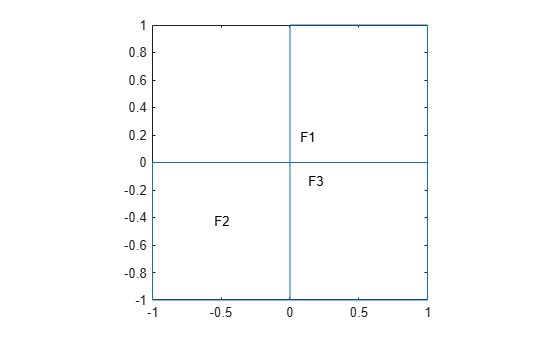# faceIC

Initial conditions on geometry face

Since R2023a

## Description

A `faceIC` object specifies the type of an initial condition on a face of a geometry. An `femodel` object contains an array of `faceIC` objects in its `FaceIC` property.

`faceIC` applies these rules for initial condition assignments:

• You can use a geometric assignment to associate the initial condition with the specified geometric regions or the entire geometry.

• You must apply a results-based assignment to all geometric regions because the `results` object contains information about the entire geometry.

• For separate assignments to a geometric region (or the entire geometry) and the boundaries of that region, the solver uses the specified assignment on the region and chooses the assignment on the boundary as follows. The solver gives an `EdgeIC` assignment precedence over a `FaceIC` assignment, even if you specify a `FaceIC` assignment after an `EdgeIC` assignment. The assignments in order of precedence are `VertexIC` (highest precedence), `EdgeIC`, `FaceIC`, and `CellIC` (lowest precedence).

## Creation

### Syntax

``model.FaceIC(FaceID) = faceIC(Name=Value)``
``model.FaceIC = faceIC(Name=Value)``

### Description

example

````model.FaceIC(FaceID) = faceIC(Name=Value)` creates a `faceIC` object and sets properties using one or more name-value arguments. This syntax assigns the specified structural, thermal, or electromagnetic initial condition to the specified faces of the geometry stored in the `femodel` object `model`. For example, `model.FaceIC([1 2]) = faceIC(Temperature = 25)` specifies the temperature on faces 1 and 2.```

example

````model.FaceIC = faceIC(Name=Value)` assigns the specified initial condition to an entire 2-D geometry or to all faces of a 3-D geometry. For example, `model.FaceIC = faceIC(Temperature = 25)` specifies the initial temperature on all faces.```

### Input Arguments

expand all

Face IDs, specified as a vector of positive integers. Find the face IDs using `pdegplot` with the `FaceLabels` value set to `"on"`.

Data Types: `double`

## Properties

expand all

Initial displacement, specified as a numeric vector, function handle, `StaticStructuralResults` object, or `TransientStructuralResults` object created by using `solve`. A numeric vector must contain two elements for a 2-D model and three elements for a 3-D model. The elements represent the components of initial displacement.

Use a function handle to specify spatially varying initial displacement. The function must return a two-row matrix for a 2-D model and a three-row matrix for a 3-D model. Each column of the matrix corresponds to the initial displacement at the coordinates provided by the solver. For details, see Nonconstant Parameters of Finite Element Model.

When you specify initial displacement as a `StaticStructuralResults` or `TransientStructuralResults` object, `faceIC` applies the results to the entire geometry. For `TransientStructuralResults`, you can access results for a particular time-step by using the `filterByIndex` function.

Initial velocity, specified as a numeric vector, function handle, `StaticStructuralResults` object, or `TransientStructuralResults` object created by using `solve`. A numeric vector must contain two elements for a 2-D model and three elements for a 3-D model. The elements represent the components of initial velocity.

Use a function handle to specify spatially varying initial velocity. The function must return a two-row matrix for a 2-D model and a three-row matrix for a 3-D model. Each column of the matrix corresponds to the initial velocity at the coordinates provided by the solver. For details, see Nonconstant Parameters of Finite Element Model.

When you specify initial velocity as a `StaticStructuralResults` or `TransientStructuralResults` object, `faceIC` applies the results to the entire geometry. For `TransientStructuralResults`, you can access results for a particular time-step by using the `filterByIndex` function.

Initial temperature or initial guess for temperature, specified as a real number, function handle, `SteadyStateThermalResults` object, or `TransientThermalResults` object. Use a function handle to specify spatially varying initial temperature. For details, see Nonconstant Parameters of Finite Element Model.

When you specify initial temperature as a `SteadyStateThermalResults` or `TransientThermalResults` object, `faceIC` applies the results to the entire geometry. For `TransientThermalResults`, you can access results for a particular time-step by using the `filterByIndex` function.

Initial guess for magnetic potential in a nonlinear magnetostatic problem, specified as a positive number, function handle, or `MagnetostaticResults` object. Use a function handle to specify spatially varying initial magnetic potential. For details, see Nonconstant Parameters of Finite Element Model.

Initial flux density, specified as a positive number or a function handle. Use a function handle to specify an initial flux density that depends on the coordinates, magnetic potential and its gradients, and the norm of magnetic flux density. For details, see Nonconstant Parameters of Finite Element Model.

If a relative permeability, current density, or magnetization for the model depend on the magnetic potential or its gradients (`state.u`, `state.ux`, and so on), then initial conditions must not depend on the magnetic flux density (`state.NormFluxDensity`).

## Examples

collapse all

Specify initial flux density for an `femodel` object representing a nonlinear magnetostatic problem.

Create an `femodel` object for solving a magnetostatic problem, and assign the L-shaped membrane geometry to the model.

```model = femodel(AnalysisType="magnetostatic", ... Geometry=@lshapeg);```

Plot the geometry with the face labels.

`pdegplot(model.Geometry,FaceLabels="on");`Specify the initial flux density for the entire geometry.

```model.FaceIC = faceIC(NormFluxDensity=1.5); model.FaceIC```
```ans = 1x3 faceIC array Properties for analysis type: magnetostatic Index MagneticVectorPotential NormFluxDensity 1 [] 1.5000 2 [] 1.5000 3 [] 1.5000 Show all properties ```

Specify the initial flux density on face 1.

```model.FaceIC(1) = faceIC(NormFluxDensity=5); model.FaceIC```
```ans = 1x3 faceIC array Properties for analysis type: magnetostatic Index MagneticVectorPotential NormFluxDensity 1 [] 5 2 [] 1.5000 3 [] 1.5000 Show all properties ```

Specify an initial temperature that depends on coordinates.

Create a rectangular geometry.

`gm = decsg([3 4 -1.5 1.5 1.5 -1.5 0 0 .2 .2]');`

Create an `femodel` object for solving a transient thermal problem, and assign the geometry to the model.

```model = femodel(AnalysisType="thermalTransient", ... Geometry=gm);```

Set the initial temperature in the rectangle to be dependent on the y-coordinate, for example, ${10}^{3}\left(0.2-{\mathit{y}}^{2}\right)$.

```T0 = @(location)10^3*(0.2 - location.y.^2); model.FaceIC = faceIC(Temperature=T0); model.FaceIC```
```ans = 1x1 faceIC array Properties for analysis type: thermalTransient Index Temperature 1 @(location)10^3*(0.2-location.y.^2) Show all properties ```

## Version History

Introduced in R2023a